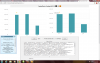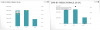## Recommended Posts

This isn't as much a problem with JS as it is an inconsistency between what's happening in the Flash and JS charts.

We have a line bar chart that is showing the numbers rounded when viewing on a PC (ie, using Flash), but not rounded when viewed through an iPad (ie, JS). For example, one of the data plots will show a tooltip value of "9/5, \$13” when viewed with Flash, but shows “9/5, \$13.039392020335924" when viewed with JavaScript (on an iPad). This is with the Round Numbers setting off. The #s get rounded in both views when the setting is set on, but this then rounds other #s that aren't desired to be rounded.

Here is the XML. Let me know if this can be fixed.

<chart showBorder="0" adjustDiv="1" alternateHGridColor="FFFFFF" animation="1" baseFont="" baseFontColor="" baseFontSize="" bgAlpha="0" bgColor="0" borderColor="FFFFFF" canvasBorderAlpha="10" canvasBorderThickness="1" canvasPadding="0" chartBottomMargin="0" chartLeftMargin="0" chartRightMargin="0" chartTopMargin="20" Decimals="0" divLineColor="CCCCCC" divLineDecimalPrecision="1" FAKECategoryFormat="" forceDecimals="0" forceSYAxisValueDecimals="" forceYAxisValueDecimals="0" formatNumberScale="0" labelDisplay="AUTO" labelPadding="" legendBgAlpha="" legendBorderAlpha="" legendNumColumns="1" legendPadding="" legendPosition="right" limitsDecimalPrecision="1" minimiseWrappingInLegend="1" numberPrefix="{:content:}quot; numberSuffix="" outCnvBaseFont="" outCnvBaseFontColor="" outCnvBaseFontSize="" palletteColors="" pFormatNumberScale="" plotFillAlpha="100" plotFillAngle="90" plotGradientColor="" PYAxisMaxValue="" PYAxisMinValue="" PYAxisName="" rotateLabels="" SAxisMaxValue="" sDecimals="0" sFormatNumberScale="" showDivLineSecondaryValue="0" showDivLineValues="1" showLegend="0" showLimits="1" showPlotBorder="0" showSecondaryLimits="0" showValues="0" sNumberPrefix="{:content:}quot; sNumberSuffix="" SYAxisMinValue="" SYAxisName="" useEllipsesWhenOverflow="" useRoundEdges="" yAxisValueDecimals="0"><categories font="Arial" fontColor="439EAA"><category label="9/5"/><category label="9/12"/><category label="9/19"/></categories><dataset showValues="0" color="439EAA" seriesname="" parentYAxis="P" alpha="100" FAKEDisplayFormat="0"><set showValues="0" displayValue="236167" value="236166.7300" Color=""/><set showValues="0" displayValue="244348" value="244348.0600" Color=""/><set showValues="0" displayValue="107687" value="107686.5400" Color=""/></dataset><dataset borderColor="FFFFFF" showValues="0" color="" seriesname="" parentYAxis="S" Thickness="4" anchorBgColor="439EAA" anchorBorderThickness="4" lineThickness="4" alpha="1" FAKEDisplayFormat="{:content:}quot; slantLabels="0"><set showValues="0" displayValue="{:content:}quot; value="13.03939202033592483128" Color=""/><set showValues="0" Color=""/><set showValues="0" Color=""/></dataset><trendLines/><ChartTitle chart_title="CPM by Week (Female 25-54)"/></chart>

Thanks,

Emilio

##### Share on other sitesHi,

Could you please confirm the chart type(swf) and the FusionCharts version you are using?

##### Share on other sites

Sure. We're using FusionCharts 3.2.1, and that particular chart was a 2D Dual Y Combination.

Thanks,

Emilio

##### Share on other sitesHi,

I am afraid, we are unable to replicate the issue, as the code is working fine from our end.##### Share on other sites

[Edit: I didn't attach properly the first time, and forgot the XML!]

That's interesting. I'm assuming the chart on the right is not using Flash. I should've grabbed a screenshot last time to accompany the XML.

I once again grabbed the XML, and a screenshot from both a PC and an iPhone. Since the last time I posted, I set Round Numbers to Yes so that the tooltip would actually show the numbers rounded. But I've set that back to No in order to recreate this scenario. Some of the data has changed, but most of the settings should be the same from the last time.

<chart showBorder="0" adjustDiv="1" alternateHGridColor="FFFFFF" animation="1" baseFont="" baseFontColor="" baseFontSize="" bgAlpha="0" bgColor="0" borderColor="FFFFFF" canvasBorderAlpha="10" canvasBorderThickness="1" canvasPadding="0" chartBottomMargin="0" chartLeftMargin="0" chartRightMargin="0" chartTopMargin="20" Decimals="0" divLineColor="CCCCCC" divLineDecimalPrecision="1" FAKECategoryFormat="" forceDecimals="0" forceSYAxisValueDecimals="0" forceYAxisValueDecimals="0" formatNumberScale="0" labelDisplay="AUTO" labelPadding="" legendBgAlpha="" legendBorderAlpha="" legendNumColumns="1" legendPadding="" legendPosition="right" limitsDecimalPrecision="1" minimiseWrappingInLegend="1" numberPrefix="\$" numberSuffix="" outCnvBaseFont="" outCnvBaseFontColor="" outCnvBaseFontSize="" palletteColors="" pFormatNumberScale="" plotFillAlpha="100" plotFillAngle="90" plotGradientColor="" PYAxisMaxValue="" PYAxisMinValue="" PYAxisName="" rotateLabels="" SAxisMaxValue="" sDecimals="0" sFormatNumberScale="" showDivLineSecondaryValue="1" showDivLineValues="1" showLegend="0" showLimits="1" showPlotBorder="0" showSecondaryLimits="1" showValues="0" sNumberPrefix="\$" sNumberSuffix="" SYAxisMinValue="" SYAxisName="" useEllipsesWhenOverflow="" useRoundEdges="" yAxisValueDecimals="0"><categories font="Arial" fontColor="439EAA"><category label="9/5"/><category label="9/12"/><category label="9/19"/></categories><dataset showValues="0" color="439EAA" seriesname="" parentYAxis="P" alpha="100" FAKEDisplayFormat="0"><set showValues="0" displayValue="236167" value="236166.7300" Color=""/><set showValues="0" displayValue="243743" value="243743.1100" Color=""/><set showValues="0" displayValue="107687" value="107686.5400" Color=""/></dataset><dataset borderColor="FFFFFF" showValues="0" color="" seriesname="" parentYAxis="S" Thickness="4" anchorBgColor="439EAA" anchorBorderThickness="4" lineThickness="4" alpha="1" FAKEDisplayFormat="\$" slantLabels="0"><set showValues="0" displayValue="\$" value="12.73081793282118982533" Color=""/><set showValues="0" displayValue="\$" value="15.68629799338421736836" Color=""/><set showValues="0" Color=""/></dataset><trendLines/><ChartTitle chart_title="CPM by Week (Female 25-54)"/></chart>

Adding to my original post, using the Round Numbers setting, at the chart level, is a compromise as then the primary y-axis numbers become abbreviated. For example, \$200,000 becomes \$200K. We'd want the default rounding to happen in both the Flash and JS renderings.

Let me know how this works on your end.

Much appreciated,

EmilioEdited by Emilio

##### Share on other sitesHi,

The code is working fine from our end, screenshot attached.

Hope this helps.##### Share on other sites

Perhaps I'm missing something, but that link takes me to a Download Trial page, prompting me for my info. Is this what I should be doing?

Thanks,

Emilio

##### Share on other sites

Hi Emilio,

Thank you for the response.

I hope this helps.

Perhaps I'm missing something, but that link takes me to a Download Trial page, prompting me for my info. Is this what I should be doing?

Thanks,

Emilio